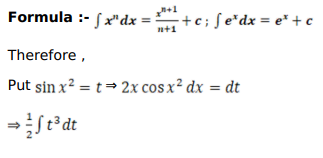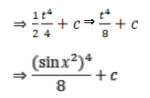# Mark against the correct answer in each of the following:

Question:

Mark $(\sqrt{)}$ against the correct answer in each of the following:

$\int x \sin ^{3} x^{2} \cos x^{2} d x=?$

A. $\frac{1}{4} \sin ^{4} x^{2}+C$

B. $\frac{1}{8} \sin ^{4} x^{2}+C$

C. $\frac{1}{2} \sin ^{4} x^{2}+C$

D. none of these

Solution: